6.2.21 CSP

6.2.21.1 Outline of the node

This estimates a sound’s direction in the horizontal plane using the CSP method from 2ch waveform data.

6.2.21.2 Necessary file

No files are required.

6.2.21.3 Usage

When to use

This node estimates a sound’s direction using the CSP method. The orientation result outputted from this node is used for post-processing such as tracking and source separation.

Typical connection

Figure 6.51 shows a typical connection example.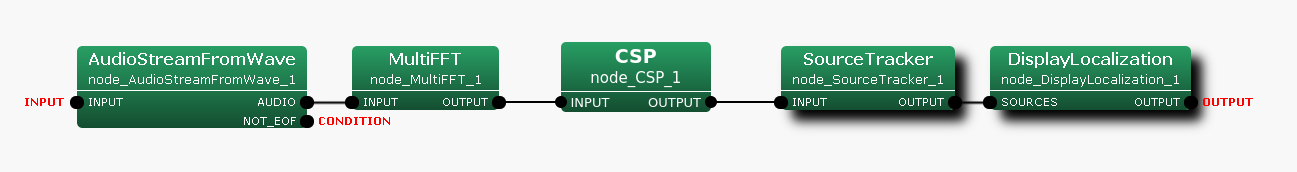Figure 6.51: Connection example of CSP

6.2.21.4 Input-output and property of the node

Input

INPUT

: Matrix<complex<float> > , Complex frequency representation of input signals with size $M \times (NFFT/2+1)$.

Output

OUTPUT

: Source position (direction) is expressed as Vector<ObjectRef>  type. ObjectRef  is a Source  and is a structure which consists of CSP value of the source and its direction. The element number of Vector  is a sound number ($N$).

CSPVALUE

: Vector<float>  type. CSP value for every direction. The output is equivalent to ${CSP_{i,j}}(k)$ in Eq.(29). This output terminal is not displayed by default.

Refer to Figure 6.52 for the addition method of hidden output.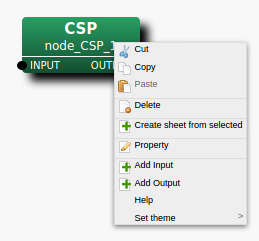Step 1: Right-click CSP  and click Add Output.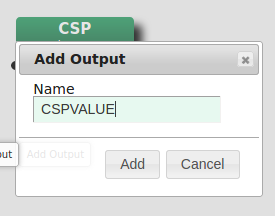Step 2: Enter CSPVALUE in the input, then, click Add.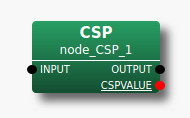Step 3: The CSPVALUE output terminal is added to the node.
Figure 6.52: Usage example of hidden outputs : Display of CSPVALUE terminal

Parameter

Table 6.38: Parameter list of CSP
 Parameter name Type Default value Unit description DISTANCE_BETWEEN_MICS 0.3 [m] Distance between microphones SAMPLING_RATE 16000 [Hz] Sampling rate SPEED_OF_SOUND 340 [m/s] Speed of sound LENGTH 512 [pt] FFT points ($NFFT$) LOWER_BOUND_FREQUENCY 500 [Hz] Lower bound frequency UPPER_BOUND_FREQUENCY 2800 [Hz] Upper bound frequency MANUAL_WEIGHT_SQUARE See below. Key point of rectangular weight MIN_DEG 0 [deg] Minimum azimuth MAX_DEG 180 [deg] Maximum azimuth WINDOW 50 [frame] Frames to normalize CrossSpectrum WINDOW_TYPE FUTURE Frame selection to normalize CrossSpectrum PERIOD 50 [frame] The cycle to compute SSL CSP_THRESHOLD 0 Threshold value of CSP value MAXNUM_OUT_PEAKS -1 Max. num. of output peaks DEBUG false ON/OFF of debug output
DISTANCE_BETWEEN_MICS

: float  type. 0.3 is default value. The distance between 2 microphones.

SAMPLING_RATE

: int  type. 16000 is the default value. Sampling frequency of input acoustic signal. It is necessary to align with other nodes like LENGTH.

SPEED_OF_SOUND

: float  type. 340 is default value. The speed of sound.

LENGTH

: int  type. 512 is the default value. FFT point in the case of fourier transform. It is necessary to align it with the FFT points to the preceding paragraph.

LOWER_BOUND_FREQUENCY

: int  type. 500 is the default value. It is the minimum of frequency bands which is taken into consideration for peak detection, and is expressed as $\omega _{min}$ in the node details. It should be $0 \leq \omega _{min} \leq {\rm SAMPLING\_ RATE} / 2$.

UPPER_BOUND_FREQUENCY

: int  type. 2800 is the default value. It is the maximum of frequency bands Which is taken into consideration for peak detections, and, is expressed as $\omega _{max}$ below. It should be $\omega _{min} < \omega _{max} \leq {\rm SAMPLING\_ RATE} / 2$.

MANUAL_WEIGHT_SQUARE

: Vector<float>  type. <Vector<float> 0.0 2000.0 4000.0 6000.0 8000.0> is the default value. By the frequency specified in MANUAL_WEIGHT_SQUARE, the rectangular weight is generated and is given to Cross spectrum. For the frequency bands from the odd components of MANUAL_WEIGHT_SQUARE to the even components, the weight of 1 is given, and for the frequency bands from the even components to the odd components, the weight of 0 is given. By default, the Cross spectrum from 2000 [Hz] to 4000 [Hz] and 6000 [Hz] to 8000 [Hz] can be suppressed.

MIN_DEG

: int  type. 0 is the default value. It is the minimum angle for peak search.

MAX_DEG

: int  type. 180 is the default value. It is the maximum angle for peak search.

WINDOW

: int  type. 50 is the default value. The number of smoothing frames for correlation matrix calculation is designated. Within the node, the correlation matrix is generated for every frame from the complex spectrum of the input signal, and the addition mean is taken by the number of frames specified in WINDOW. Although the correlation matrix will be stabilized if this value is enlarged, time delays become long due to the long interval.

WINDOW_TYPE

: string  type. FUTURE is the default value. The selection of used smoothing frames for correlation matrix calculation. Let $f$ be the current frame. If FUTURE, frames from $f$ to $f+WINDOW-1$ will be used for the normalization. If MIDDLW, frames from $f-(WINDOW/2)$ to $f+(WINDOW/2)+(WINDOW\% 2)-1$ will be used for the normalization. If PAST, frames from $f-WINDOW+1$ to $f$ will be used for the normalization.

PERIOD

: int  type. 50 is the default value. The cycle of SSL calculation is specified in frames number. If this value is large, the time interval for obtaining the orientation result becomes large, which will result in improper acquisition of the speech interval or bad tracking of the mobile sound. However, since the computational cost increases if it is small, tuning according to the computing environment is needed.

CSP_THRESHOLD

: float  type. 0 is default value. This node pick up the local-peak from CSP value which is larger than this value.

MAXNUM_OUT_PEAKS

: int type. -1 is the default. This parameter defines the maximum number of output peaks of CSP value (sound sources). If -1 or 0, all the peaks are output. If MAXNUM_OUT_PEAKS $> 0$, MAXNUM_OUT_PEAKS peaks are output in order of their value.

DEBUG

: bool  type. ON/OFF of the debug output and the format of the debug output is CSP value.

6.2.21.5 Details of the node

CSP method estimates the sound’s direction from CSP value and Time Difference of Arrival (TDOA), which are calculated from 2ch signales ($s_{i}(n)$ , $s_{j}(n)$) recording with 2 microphones ($M_{i}$ , $M_{j}$) . CSP value and TDOA are expressed as follows.

 \begin{equation} \label{eq:CSP-value} CSP_{i,j}(k) = DFT^{-1}[\frac{DFT[s_{i}(n)]DFT[s_{j}(n)]^{\ast }}{|DFT[s_{i}(n)]||DFT[s_{j}(n)]|}] \end{equation} (29)
 \begin{equation} \label{eq:CSP-TDOA} \tau = argmax_{k}(CSP_{i,j}(k)) \end{equation} (30)

$\tau$ is the time (samples) difference of the sound, and CSP value has a local peak at the time. The sound’s direction is expressed as follows with the time differenct $\tau$, the spped of sound $c$, the distance between 2 microphones and the sampling rate $F_{s}$.

 \begin{equation} \label{eq:CSP-theta} \theta = \cos ^{-1}(\frac{c \tau / F_{s}}{d}) \end{equation} (31)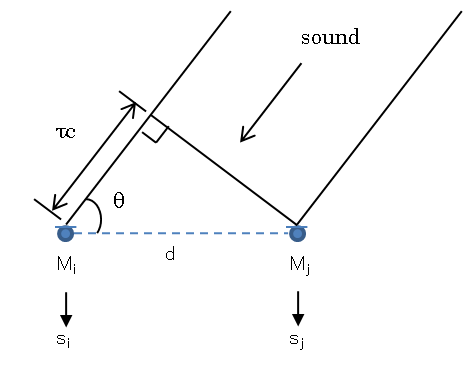Figure 6.53: CSP method

6.2.21.6 References

• Shun Tsunasawa, Shinji Ohyama, “Multi-speaker Localization and Tracking Based on TDOA Derived from Multi-frame CSP Coefficient” Transactions of the Society of Instrument and Control Engineers, Vol.53, No.12, 644/653 (2017).